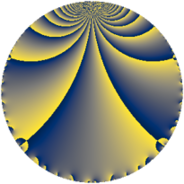# Properties

 Label 6080.2.a.lLevel $6080$ Weight $2$ Character orbit 6080.a Self dual yes Analytic conductor $48.549$ Analytic rank $1$ Dimension $1$ CM no Inner twists $1$

# Related objects

Show commands: Magma / PariGP / SageMath

## Newspace parameters

comment: Compute space of new eigenforms

[N,k,chi] = [6080,2,Mod(1,6080)]

mf = mfinit([N,k,chi],0)

lf = mfeigenbasis(mf)

from sage.modular.dirichlet import DirichletCharacter

H = DirichletGroup(6080, base_ring=CyclotomicField(2))

chi = DirichletCharacter(H, H._module([0, 0, 0, 0]))

N = Newforms(chi, 2, names="a")

//Please install CHIMP (https://github.com/edgarcosta/CHIMP) if you want to run this code

chi := DirichletCharacter("6080.1");

S:= CuspForms(chi, 2);

N := Newforms(S);

 Level: $$N$$ $$=$$ $$6080 = 2^{6} \cdot 5 \cdot 19$$ Weight: $$k$$ $$=$$ $$2$$ Character orbit: $$[\chi]$$ $$=$$ 6080.a (trivial)

## Newform invariants

comment: select newform

sage: f = N # Warning: the index may be different

gp: f = lf \\ Warning: the index may be different

 Self dual: yes Analytic conductor: $$48.5490444289$$ Analytic rank: $$1$$ Dimension: $$1$$ Coefficient field: $$\mathbb{Q}$$ Coefficient ring: $$\mathbb{Z}$$ Coefficient ring index: $$1$$ Twist minimal: no (minimal twist has level 3040) Fricke sign: $$1$$ Sato-Tate group: $\mathrm{SU}(2)$

## $q$-expansion

comment: q-expansion

sage: f.q_expansion() # note that sage often uses an isomorphic number field

gp: mfcoefs(f, 20)

 $$f(q)$$ $$=$$ $$q + q^{5} - 2 q^{7} - 3 q^{9}+O(q^{10})$$ q + q^5 - 2 * q^7 - 3 * q^9 $$q + q^{5} - 2 q^{7} - 3 q^{9} - 4 q^{11} + 4 q^{13} + 6 q^{17} - q^{19} + 6 q^{23} + q^{25} - 6 q^{29} - 8 q^{31} - 2 q^{35} + 4 q^{37} + 6 q^{41} + 2 q^{43} - 3 q^{45} - 10 q^{47} - 3 q^{49} - 4 q^{55} + 4 q^{59} + 2 q^{61} + 6 q^{63} + 4 q^{65} + 16 q^{67} - 8 q^{71} - 2 q^{73} + 8 q^{77} - 16 q^{79} + 9 q^{81} + 6 q^{83} + 6 q^{85} - 10 q^{89} - 8 q^{91} - q^{95} - 12 q^{97} + 12 q^{99}+O(q^{100})$$ q + q^5 - 2 * q^7 - 3 * q^9 - 4 * q^11 + 4 * q^13 + 6 * q^17 - q^19 + 6 * q^23 + q^25 - 6 * q^29 - 8 * q^31 - 2 * q^35 + 4 * q^37 + 6 * q^41 + 2 * q^43 - 3 * q^45 - 10 * q^47 - 3 * q^49 - 4 * q^55 + 4 * q^59 + 2 * q^61 + 6 * q^63 + 4 * q^65 + 16 * q^67 - 8 * q^71 - 2 * q^73 + 8 * q^77 - 16 * q^79 + 9 * q^81 + 6 * q^83 + 6 * q^85 - 10 * q^89 - 8 * q^91 - q^95 - 12 * q^97 + 12 * q^99

## Embeddings

For each embedding $$\iota_m$$ of the coefficient field, the values $$\iota_m(a_n)$$ are shown below.

For more information on an embedded modular form you can click on its label.

comment: embeddings in the coefficient field

gp: mfembed(f)

Label $$\iota_m(\nu)$$ $$a_{2}$$ $$a_{3}$$ $$a_{4}$$ $$a_{5}$$ $$a_{6}$$ $$a_{7}$$ $$a_{8}$$ $$a_{9}$$ $$a_{10}$$
1.1
 0
0 0 0 1.00000 0 −2.00000 0 −3.00000 0
 $$n$$: e.g. 2-40 or 990-1000 Significant digits: Format: Complex embeddings Normalized embeddings Satake parameters Satake angles

## Atkin-Lehner signs

$$p$$ Sign
$$2$$ $$-1$$
$$5$$ $$-1$$
$$19$$ $$1$$

## Inner twists

This newform does not admit any (nontrivial) inner twists.

## Twists

By twisting character orbit
Char Parity Ord Mult Type Twist Min Dim
1.a even 1 1 trivial 6080.2.a.l 1
4.b odd 2 1 6080.2.a.o 1
8.b even 2 1 3040.2.a.b 1
8.d odd 2 1 3040.2.a.c yes 1

By twisted newform orbit
Twist Min Dim Char Parity Ord Mult Type
3040.2.a.b 1 8.b even 2 1
3040.2.a.c yes 1 8.d odd 2 1
6080.2.a.l 1 1.a even 1 1 trivial
6080.2.a.o 1 4.b odd 2 1

## Hecke kernels

This newform subspace can be constructed as the intersection of the kernels of the following linear operators acting on $$S_{2}^{\mathrm{new}}(\Gamma_0(6080))$$:

 $$T_{3}$$ T3 $$T_{7} + 2$$ T7 + 2 $$T_{11} + 4$$ T11 + 4

## Hecke characteristic polynomials

$p$ $F_p(T)$
$2$ $$T$$
$3$ $$T$$
$5$ $$T - 1$$
$7$ $$T + 2$$
$11$ $$T + 4$$
$13$ $$T - 4$$
$17$ $$T - 6$$
$19$ $$T + 1$$
$23$ $$T - 6$$
$29$ $$T + 6$$
$31$ $$T + 8$$
$37$ $$T - 4$$
$41$ $$T - 6$$
$43$ $$T - 2$$
$47$ $$T + 10$$
$53$ $$T$$
$59$ $$T - 4$$
$61$ $$T - 2$$
$67$ $$T - 16$$
$71$ $$T + 8$$
$73$ $$T + 2$$
$79$ $$T + 16$$
$83$ $$T - 6$$
$89$ $$T + 10$$
$97$ $$T + 12$$